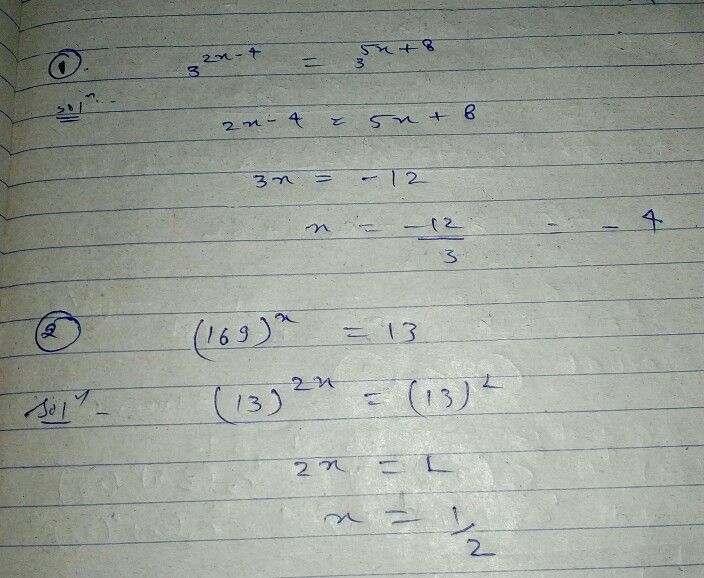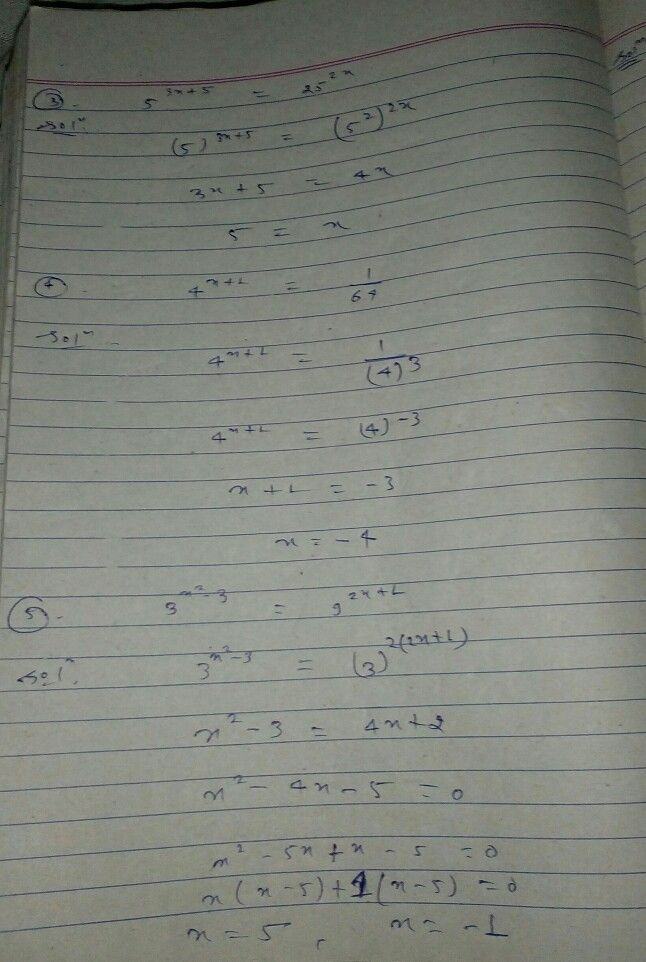Symbol
ProblemActivity $2$ $S$ Solve for the values of $x$ for each of the following exponential equations. $1$ $3^{2x-4}=3^{5x+8}$ $2$ $169^{x}=13$ $3$ $53x+8=252x$ $4$ $4x+1=\dfrac {1} {64}$ $5.$ $3^{x^{2}-3}=92x+1$
10th-13th grade
Other
Search count: 115
SolutionQanda teacher - HARSHITStudent
thank you so muchQanda teacher - HARSHIT
thanks
hope you satisfied by me